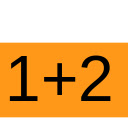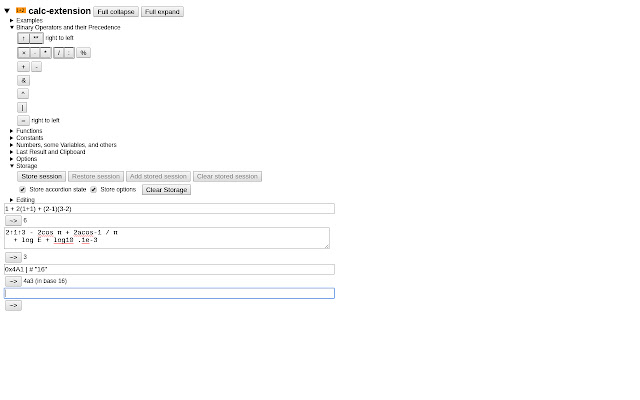# calc-extension

CRX id

## manfffpjhdpdkeinlfcanehifhakepcjDescription from extension meta

## Calculate values of mathematical expressions

Image from storeDescription from store # calc-extension (C) Martin Väth <[email protected]> This project is under the GNU Public License 2.0. A WebExtension: Calculate values of mathematical expressions The extension strives to be useful for everybody quickly needing brief or mid-long calculations: in daily “browser” life, as a mathematician, or as a programmer - there is support for all needs. Formulas can be entered in the typical mathematician's or programmer's syntax - both is understood. There is also support for lengthy sessions (saving/restoring, exporting to clipboard, etc). The original motivation was to experiment with a Pratt parser. There is already a much richer expression syntax than in most languages (implicit multiplication signs, no braces necessary for function calls), and it would be simple to extend the syntax to a more full-blown “language”. (In fact, earlier versions of __calc-extension__ had a simpler syntax. Readers interested in the parser implementation can check `data/js/parser.js`). After installing __calc-extension__, it can be used as follows. Click the __calc-extension__ symbol `1+2` or the link on the options page. Then a page opens where you can enter a formula (see below) which will be calculated when you press the solver button (`~>`). (There is an option to use the enter key instead if you prefer.) The result is then shown and you can enter the next formula. You can also go back to an earlier entered formula and “recalculate” it (e.g. after you modified some variables). To remove a formula from your list, simply remove the main formula text and press the solver button. A simple formula is a usual mathematical expression which consists of numbers, the usual operators `+` `-` `*` `/` and braces `(` `)`. As usual in mathematics (though not in most computer languages), it is possible to omit the multiplication sign `*`. However: __Be aware that `x` means the variable x and not a multiplication sign__! For multiplication use instead one of the symbols `*` `·` `×` or simply omit it (a space can be used to separate adjacent tokens). The division symbol is `/` or `:`. Numbers have the usual floating-point format, e.g. `17` `0.1` `1.2e-3`, or they can be octal or hexadecimal: For an octal number start with `0`, for a hexadecimal number start with `0x`. There are further operators which can be used in expressions: - `%` remainder (“modulo”) - `**`or `↑` exponentiation (binds from right to left) - `&` bitwise AND - `|` bitwise OR - `^` bitwise XOR There are also some mathematical functions available. Note that functions bind stronger than all binary operators, i.e. `sin PI/2` is the same as `sin(PI)/2` but differs from `sin(PI/2)`. - `sin` the sine, argument is in radians - `cos` the cosine, argument is in radians - `tan` the tangent, argument is in radians - `asin` the arcsine, in radians - `acos` the arccosine, in radians - `atan` the arctangent, in radians - `sinh` the hyperbolic sine - `cosh` the hyperbolic cosine - `tanh` the hyperbolic tangent - `asinh` the hyperbolic areasine - `acosh` the hyperbolic areacosine - `atanh` the hyperbolic areatangent - `log10` the base 10 logarithm - `log2` the base 2 logarithm - `log` the natural logarithm (base E) - `log1p` `log(1+x)` where `x` is the argument - `exp` the exponential function (base E) - `expm1` `exp x - 1` where `x` is the argument - `sqrt` the square root, that is `sqrt x = x ** (1/2)` - `cbrt` the cube root, that is `cbrt x = x ** (1/3)` - `abs` the absolute value - `sign` the signum (1, 0, or -1) - `floor` the value rounded down to an integer - `ceil` the value rounded up to an integer - `trunc` the value rounded to the integer of smaller absolute value - `round` the value rounded to its nearest integer - `fround` the value rounded to its nearest 32 bit float - `clz32` the number of leading zero bits in a 32 bit representation Furthermore, there are constants available: - `E` Euler's number exp 1 - `PI` or `π` the circle number acos -1 - `SQRT2` sqrt 2 - `SQRT1_2` sqrt 1/2 - `LN2` log 2 - `LN10` log 10 - `LOG2E` log2 E - `LOG10E` log10 E - `EPSILON` or `ε` the distance of 1 to the smallest larger floating point number Finally, it is possible to define variables with e.g. `a=...` and to use them. Variable names must only consist of English characters, numbers, or `_`. The names of the functions and constants and operator precedence is similar to that of javascript. However, functions in javascript require braces, and the multiplication sign must not omitted. The following things are further extensions: There is a special variable name `#` which always refers to the result of the last (succesful) calculation. There are also special sequences which can occur anywhere in an expression and which cause options to switch (you can also switch them by the mouse). To modify options more permanently (also for future sessions even across browser restarts), you have to store them or use the preferences window. - `'width:height'` textarea size - `"base"` switch output to base (2-36) - `!` new input fields are textareas (multiple lines) - `?` new input fields are for single lines The actual calculation of the functions and number conversion occurs by javascript calls. Therefore, mathematical properties like available precision, error messages, number limitations, and possible inaccuracies (and possibly even bugs) are inherited from the javascript interpreter. ## Example session - `1 + 2(1+1) + (2-1)(3-2)` - ~> 6 - `1·1 + 1×1 + 1 1 + 1*1` - ~> 4 - `2↑2↑3 - 2**8 + 4/3 - 1:3` - -> 1 - `1 - cos π/2 + cos(PI/2)` - ~> 1.5 - `a = 3(# + 1)` - ~> 7.5 - `# + 4a` - ~> 37.5 - `0xF | 0100 "16"` - ~> 4f (in base 16) ## Permissions The extension requires the storage permission in order to store options/accordion state and possibly a session on local storage. ## Languages Currently, the following languages are supported: - en (default language) - de

### Statistics

Installs
58 history
Category
Rating
0.0 (0 votes)
Last update / version
2020-09-03 / 5.4
Listing languages